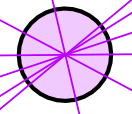# Activity: Symmetry of Shapes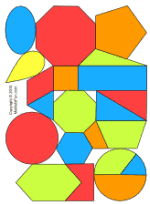Let's find symmetry in shapes! You will need some shapes. You could buy some, or make your own like this: Print out Shapes (In Color) or Shapes (black and white) and cut them out.

## Folding Test

You can find if a shape has a Line of Symmetry by folding it.

When the folded part sits perfectly on top (all edges matching), then the fold line is a Line of Symmetry.

Here I have folded a rectangle one way, and it didn't work.So this is not a Line of Symmetry

But when I try it this way, it does work (the folded part sits perfectly on top, all edges matching):So this is a Line of Symmetry

## An Octagon

Let us try the Octagon (the 8-sided shape)

Is this a Line of Symmetry?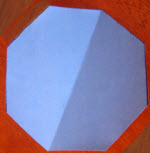Let's try folding it:Yes! When folded over, the edges match perfectly

So let us draw it on: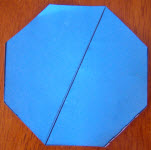I found another way too::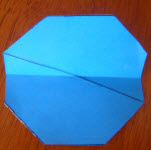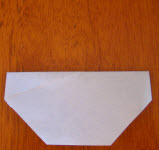Tried this It works!

So let us draw it on, too:In fact I found 8 Lines of Symmetry: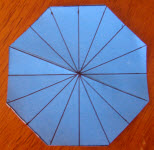## Triangles

 How about this Triangle?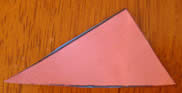I tried this fold, but it didn't work: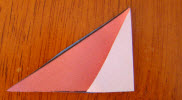Can you find any Lines of Symmetry in that triangle? I couldn't.

But how about other types of triangle?

Now it is your turn ... pick a shape and find its Lines of Symmetry.

In fact, try them all! See what you discover.

## Final Note: The Circle

How about the Circle? Did you find any Lines of Symmetry?

In fact the circle has infinite Lines of Symmetry, how about that!